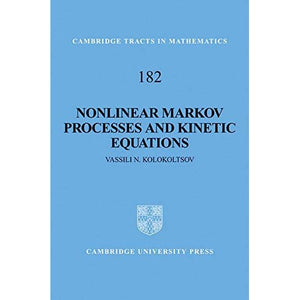># Nonlinear Markov Processes and Kinetic Equations (Cambridge Tracts in Mathematics)

• £32.89
• Save £34

Vassili N. Kolokoltsov
Cambridge University Press, 8/28/2010
EAN 9780521111843, ISBN10: 0521111846

Hardcover, 400 pages, 23.6 x 16 x 2.1 cm
Language: English

A nonlinear Markov evolution is a dynamical system generated by a measure-valued ordinary differential equation with the specific feature of preserving positivity. This feature distinguishes it from general vector-valued differential equations and yields a natural link with probability, both in interpreting results and in the tools of analysis. This brilliant book, the first devoted to the area, develops this interplay between probability and analysis. After systematically presenting both analytic and probabilistic techniques, the author uses probability to obtain deeper insight into nonlinear dynamics, and analysis to tackle difficult problems in the description of random and chaotic behavior. The book addresses the most fundamental questions in the theory of nonlinear Markov processes: existence, uniqueness, constructions, approximation schemes, regularity, law of large numbers and probabilistic interpretations. Its careful exposition makes the book accessible to researchers and graduate students in stochastic and functional analysis with applications to mathematical physics and systems biology.

Preface
Basic notations
1. Introduction
Part I. Tools From Markov Processes
2. Probability and analysis
3. Probabilistic constructions
4. Analytic constructions
5. Unbounded coefficients
Part II. Nonlinear Markov Processes and Semigroups
6. Integral generators